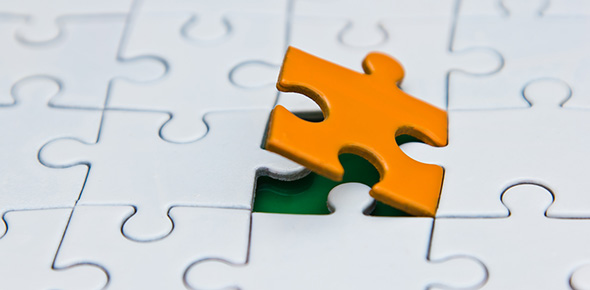# Mtca Chapter 3 Prefixes - Quiz 5

20 Questions | Total Attempts: 118Settings• 1.
As in the term diameter, the prefix “dia-” means ___.
• A.

Within, inside

• B.

Around

• C.

Half

• D.

Through, between

• 2.
In the term pericardium, the prefix “peri-” means ___.
• A.

Between

• B.

Around

• C.

Beside, alongside

• D.

Outside, beyond

• 3.
In the term intercostal, the prefix “inter-” means ___.
• A.

Middle

• B.

Before, in front of

• C.

Together, with

• D.

Between

• 4.
The prefix that means “first” is ___.
• A.

Pro-

• B.

Primi-

• C.

Uni-

• D.

Mono-

• 5.
Which prefix would be used to denote the smallest number when combined with the suffix "-meter"?
• A.

Milli-

• B.

Centi-

• C.

Hemi-

• D.

Deca-

• 6.
Someone involved in intraspection, is investigating ___.
• A.

Without

• B.

Within

• C.

Around

• D.

Beyond

• 7.
Which pair of prefixes could both be used to refer to something “above”?
• A.

Hyper- & supra-

• B.

Super- & hypo-

• C.

Supra- & pre-

• D.

Sub- & epi-

• 8.
In the terms contraception and contraband, the prefix “contra-” means ___.
• A.

Without

• B.

Away from

• C.

Against

• D.

• 9.
The prefix that could be used to mean “beyond, between, or change” is ___.
• A.

Micro-

• B.

Mega-

• C.

Meta-

• D.

Meso-

• 10.
The prefix that would be the opposite of “mega-” is ___.
• A.

Pan-

• B.

Sub-

• C.

Oligo-

• D.

Poly-

• 11.
The prefix that can mean both “down” and “away from” is ___.
• A.

Ab-

• B.

Ex-

• C.

De-

• D.

Cata-

• 12.
In the term dislocation, the prefix “dis-” has the same meaning as which of the following prefixes?
• A.

Ec-

• B.

Dys-

• C.

Cac-

• D.

Dif-

• 13.
In the term megalomania, the prefix “mega-” means ___.
• A.

Large, great

• B.

Small

• C.

All

• D.

Beyond

• 14.
In the term homogenous, the prefix “homo-” means ___.
• A.

Different

• B.

Same

• C.

Below

• D.

Above

• 15.
Based upon the prefix, the term that would best refer to the “outer” layer of the skin is ___.
• A.

Endo/derm

• B.

Ecto/derm

• C.

Peri/derm

• D.

Meso/derm

• 16.
In the term incontinence, the prefix “in-” means ___.
• A.

• B.

Out

• C.

Not

• D.

Apart

• 17.
In the term brachytherapy, the prefix “brachy-” means to ___.
• A.

Short

• B.

Poor

• C.

Fast

• D.

Slow

• 18.
The prefix that means “before” is ___.
• A.

An-

• B.

Extra-

• C.

Supra-

• D.

Pro-

• 19.
Based upon the meaning of the prefix “circum-”,  the term circumscribe means ___.
• A.

To write within

• B.

To write against

• C.

To write between

• D.

To write around

• 20.
The prefix that means “beside, alongside, abnormal” is ___.
• A.

Pseudo-

• B.

Para-

• C.

Peri-

• D.

Pan-Back to top